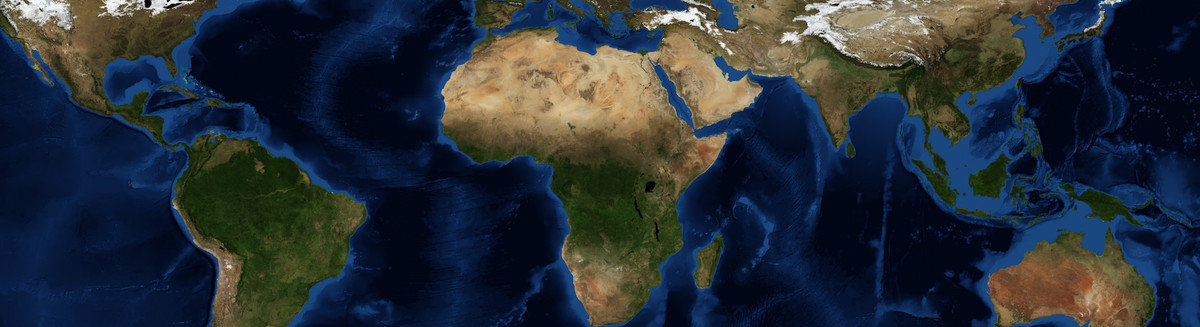This is a Preprint and has not been peer reviewed. This is version 1 of this Preprint.

##### Authors

Hao Fu, Shiwei Sun

##### Abstract

Rotating Rayleigh-Bénard convection (RRBC) denotes the free convection between two parallel plates with a fixed temperature difference, placed in a rotating reference frame. It is a prototype model of geophysical and astrophysical convection. Rotation breaks the symmetry on its rotating axis, making the cyclones and anticyclones unequal in size and magnitude. Such an asymmetry has long been observed in experiments and simulations, but has not been explained with any theoretical model. A theory of such vorticity asymmetry is proposed specifically for the cellular regime, where background rotation is important and convection is weak. The property that columnar updraft and downdraft plumes are densely packed is shown to make the vertical vorticity profile at the vortex center approximately linear with height via thermal wind relation. This simplification of morphology enables a linkage between the vorticity strength of a plume which is quantified by vorticity Rossby number $\mathrm{Ro_V}$, and the vorticity magnitude difference between the cyclonic and anticyclonic ends of plumes which is quantified with a nondimensional asymmetry factor $\delta$. The lowest order relationship between $\delta$ and $\mathrm{Ro_V}$ is found to be constrained by vertical vorticity equation alone. An approximate analytical solution is found using asymptotic expansion, which shows that the asymmetry is generated mainly by the vertical advection and stretching of vertical vorticity in fluid interior, and is modified by the Ekman layer dynamics.

##### DOI

https://doi.org/10.31223/X5N90D

##### Subjects

Physical Sciences and Mathematics

##### Keywords

convection, rotationg, symmetry breaking

##### Dates

Published: 2021-09-12 16:26

Last Updated: 2021-09-12 23:26